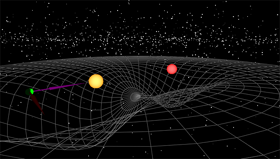A# negative mass

Negative mass is a hypothetical counterpart to ordinary (positive) mass. Although it is not known if negative mass exists, or even if its existence is theoretically possible, a number of scientists have speculated on its properties. Among these are Hermann Bondi1 in the 1950s, Banesh Hoffman (1906–1986), of the City University, New York, in the 1960s and '70s, and Robert Forward,2 in the context of spacecraft propulsion, in the 1980s. In both Newton's and Einstein's theories of gravity, negative mass is a requirement for antigravity to exist.

## Introduction

The concept of negative mass arises in the first instance by analogy with electric charges, of which there are both positive and negative varieties. Just as a positive electric charge can be canceled by a negative charge, thus giving rise to the possibility of screening against electric forces, so we can envisage the possibility of "gravity screens," if negative mass existed to neutralize ordinary, positive mass – an idea much explored by science fiction writers.

To begin to grasp the physics of negative mass, consider an ordinary mass in the absence of gravity – a spacecraft, say, that is far from the Earth, the Sun, or any other gravitating body. The mass of the spacecraft would manifest itself as inertia, in other words as resistance to any change in its motion: the more massive it is, the bigger the force needed to accelerate it or deflect it from its course. Ordinary mass moves in the direction it is pushed, with an acceleration proportional to the pushing force (as given by Newton's equation, F = m × a). Negative mass, by contrast, would presumably move toward a pushing force and away from a pulling force, with accelerations proportional to the size of these forces.

Now, let's bring gravity into consideration. Gravity is always an attractive, pulling force in its interaction with ordinary matter. Wouldn't the reverse be true in the case of negative mass? You might suppose that if a negative mass were placed near the Earth's surface it would move away from the latter's gravitational pull and effectively fall upward. However, the situation isn't quite so straightforward. The reason for this is that gravity is a mutual attraction between two masses: just as an apple falls to Earth, so Earth falls toward an apple (albeit by a much smaller amount). This reciprocity is inherent in Newton's law of gravitation, later refined, but not essentially changed, by Einstein. The law states that the gravitational attraction between two objects is proportional to the product of their masses, say m x M, where m, if we like, is the mass of the apple and M is the mass of the Earth.

Suppose now we have an apple of negative mass, -m. The gravitational force between this and the Earth is proportional to (-m) × M. That is to say it has the same strength as before but, because of the negative sign, is oppositely directed. The gravitational attraction has become a repulsion tending to push the apple away. But, from what we said earlier, a negative mass should move in the opposite direction to which it is pushed. So, a negative mass apple ought to fall downward after all!Image courtesy of www.gravitation3d.com

Since we don't currently have any objects of negative mass to experiment with, we don't know for sure if this is what would happen. However, if such objects were observed to fall upward instead then we'd have to suppose that positive and negative masses have either the same inertial properties, or the same gravitational properties, but not both. For example, we might assume that the gravitational force between two masses is proportional only to the numerical magnitude of their product, regardless of whether this product is negative or positive. Then a negative mass would be attracted by Earth's gravity but, because of its inertial perversity, would respond to this attraction by falling upward.

Such an argument would, however, lead to a serious conflict with Einstein's general theory of relativity, the beauty and power of which derive from treating the gravitational and inertial aspects of mass, not as essentially different entities, but rather as different sides of the same cosmic coin.

## Conversion of positive into negative mass

Banesh Hoffmann, a one-time collaborator of Einstein's, explored in mathematical detail some possible consequences of the existence of negative mass.3 He assumed, in accordance with relativity theory, that both positive and negative masses can radiate gravitational waves (just as electric charges can radiate electromagnetic waves); moreover relativity theory suggests that the energy carried by such waves if positive, regardless of the sign of the radiating mass. This leads to the remarkable result that a positive mass should be able to transform itself into a negative one by radiating away sufficient energy as gravitational waves and that further radiation should makes its mass increasingly negative.

## Negative mass propulsion

Negative mass propulsion is a hypothetical propulsion system based on the juxtaposition of ordinary positive mass and negative mass. In theory, such a system would be able to provide continuous thrust, without violating the principles of conservation of momentum or energy. It would require no input energy and no reaction mass. Workability of the scheme, however, hinges on the existence of negative mass and also on negative mass having negative inertia. The combined interactions of the two types of mass would then result in a sustained acceleration of both masses in the same direction.

### References

1. Bondi, H. "Negative Mass in General Relativity," Reviews of Modern Physics, Vol. 29, No.3, July 1957, pp. 423-428.
2. Forward, R. L. "Negative Matter Propulsion", Journal of Propulsion and Power (AIAA), Vol. 6, No. 1, Jan.-Feb. 1990, pp. 28-37.
3. Hoffmann, Banesh. "Negative Mass," Science Journal, April 1965, pp. 74-78.
4. Winterberg, F. "On Negative Mass Propulsion," International Astronautical Federation, Paper 89-668, 40th Congress of the International Astronautical Federation, Malaga, Spain, Oct., 1989.